### Home > A2C > Chapter 5 > Lesson 5.2.2 > Problem5-84

5-84.
1. This is a Checkpoint for solving for one variable in an equation with two or more variables.Rewrite the following equations so that you could enter them into the graphing calculator. In other words, solve for y. Homework Help ✎

1. x − 3(y + 2) = 6

2.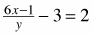3.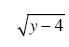= x + 1

4.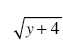= x + 2

5. Check your answers by referring to the Checkpoint 10 materials located at the back of your book.

If you needed help to solve these equations correctly, then you need more practice in solving for one variable in an equation involving two or more variables. Review Checkpoint 10 materials and try the practice problems. Also, consider getting help outside of class time. From this point on, you will be expected to solve equations such as these easily and accurately.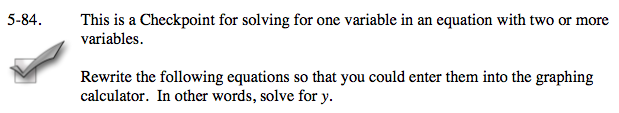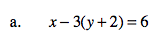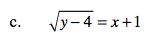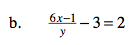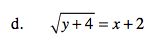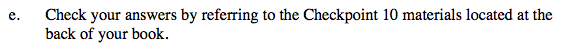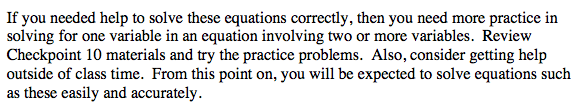Answers and extra practice are located in the back of your printed textbook or in the Reference Tab of your eBook.
If you have an eBook for A2C , login and then click the following link: Checkpoint 10 Answers and Practice